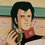# Deriving Formula for Centripetal Force

The conditions for a centripetal force to be present is circular motion, specifically when the angular frequency and speed are constant. First, start off with the vector or parametric equation for circular motion about the origin. The radius of the circular path is r meters, the angular frequency of the particle is omega.

$\mathbf{s}=\begin{pmatrix}x\\ y\end{pmatrix}=\begin{pmatrix}r \cos\left ( \omega t \right )\\ r \sin\left ( \omega t \right ) \end{pmatrix}$

The definition of velocity is the rate of change in displacement, so to get velocity, differentiate the position vector for the particle with respect to time.

$\mathbf{v}=\frac{d}{dt}\mathbf{s}=\begin{pmatrix}-r \omega \sin\left ( \omega t \right )\\ r \omega \cos\left ( \omega t\right )\end{pmatrix}$

Velocity is not constant but if we take the magnitude, we find that its magnitude (speed) is, so the conditions for centripetal force to be present are satisfied.

$|\mathbf{v}|=\sqrt{(r\omega)^2 \cos^2 \left ( \omega t \right ) +(r\omega)^2 \sin^2 \left ( \omega t \right )}$

$|\mathbf{v}|=r\omega\sqrt{\cos^2 \left ( \omega t \right ) +\sin^2 \left ( \omega t \right )}$

$\therefore |\mathbf{v}|=r\omega =r 2\pi f$

Acceleration is the rate of change in velocity so differentiate the velocity vector to get the acceleration vector.

$\mathbf{a}=\frac{d}{dt}\mathbf{v}=\begin{pmatrix}-r \omega^2 \cos\left ( \omega t \right )\\ -r \omega^2 \sin\left ( \omega t\right )\end{pmatrix}$

With this expression for acceleration, we can show that acceleration is always perpendicular to velocity, another requirement for circular motion. Velocity is perpendicular to acceleration if and only if their scalar product is zero.

$\mathbf{v}\perp\mathbf{a} \Leftrightarrow \mathbf{v}\cdot\mathbf{a}=0$

$\mathbf{v}\cdot\mathbf{a}=\begin{pmatrix}-r \omega \sin\left ( \omega t \right )\\ r \omega \cos\left ( \omega t\right )\end{pmatrix}\cdot\begin{pmatrix}-r \omega^2 \cos\left ( \omega t \right )\\ -r \omega^2 \sin\left ( \omega t\right )\end{pmatrix}=r^2\omega^3\cos \left ( \omega t \right ) \sin\left ( \omega t \right )-r^2\omega^3\cos \left ( \omega t \right ) \sin\left ( \omega t \right )=0$

$\therefore \mathbf{v}\perp\mathbf{a}$

Moving on, the magnitude of acceleration is

$|\mathbf{a}|=\sqrt{r^2 \omega^4 \sin^2 \left ( \omega t \right ) +r^2 \omega^4 \cos^2 \left ( \omega t \right )}=r \omega^2$

Multiply by $r$

$r|\mathbf{a}| =r^2 \omega^2=\left (r \omega \right ) ^2$

Substitute $|\mathbf{v}|=r\omega$

$r|\mathbf{a}| =|\mathbf{v}|^2$

Divide by $r$ and we have the formula for centripetal acceleration.

$\therefore |\mathbf{a}| =\frac{|\mathbf{v}|^2}{r}$

Assuming the particle has a constant mass, multiply both sides by $m$ and substitute the left hand side for the magnitude of the force vector because of Newton's second law. Thus we have the formula for centripetal force.

$\therefore |\mathbf{F}| =m\frac{|\mathbf{v}|^2}{r}$Note by Jack Han
5 years, 9 months ago

This discussion board is a place to discuss our Daily Challenges and the math and science related to those challenges. Explanations are more than just a solution — they should explain the steps and thinking strategies that you used to obtain the solution. Comments should further the discussion of math and science.

When posting on Brilliant:

• Use the emojis to react to an explanation, whether you're congratulating a job well done , or just really confused .
• Ask specific questions about the challenge or the steps in somebody's explanation. Well-posed questions can add a lot to the discussion, but posting "I don't understand!" doesn't help anyone.
• Try to contribute something new to the discussion, whether it is an extension, generalization or other idea related to the challenge.

MarkdownAppears as
*italics* or _italics_ italics
**bold** or __bold__ bold
- bulleted- list
• bulleted
• list
1. numbered2. list
1. numbered
2. list
Note: you must add a full line of space before and after lists for them to show up correctly
paragraph 1paragraph 2

paragraph 1

paragraph 2

[example link](https://brilliant.org)example link
> This is a quote
This is a quote
    # I indented these lines
# 4 spaces, and now they show
# up as a code block.

print "hello world"
# I indented these lines
# 4 spaces, and now they show
# up as a code block.

print "hello world"
MathAppears as
Remember to wrap math in $$ ... $$ or $ ... $ to ensure proper formatting.
2 \times 3 $2 \times 3$
2^{34} $2^{34}$
a_{i-1} $a_{i-1}$
\frac{2}{3} $\frac{2}{3}$
\sqrt{2} $\sqrt{2}$
\sum_{i=1}^3 $\sum_{i=1}^3$
\sin \theta $\sin \theta$
\boxed{123} $\boxed{123}$